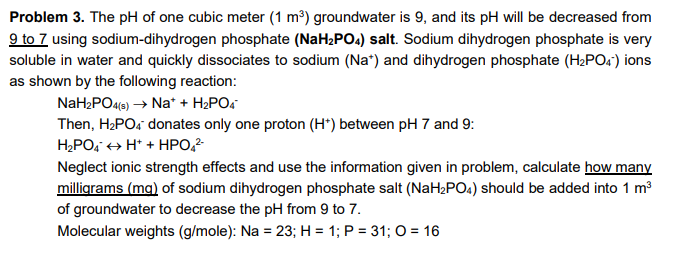### Create an Account

Home / Questions / The pH of one cubic meter (1 m2) groundwater is 9, and its pH will be decreased from 9 to ...

# The pH of one cubic meter (1 m2) groundwater is 9, and its pH will be decreased from 9 to 7 using sodium-dihydrogen phosphate (NaH2PO4) salt. Sodium dihydrogen phosphate is very soluble in water and

The pH of one cubic meter (1 m2) groundwater is 9, and its pH will be decreased from 9 to 7 using sodium-dihydrogen phosphate (NaH2PO4) salt. Sodium dihydrogen phosphate is very soluble in water and quickly dissociates to sodium (Na+) and dihydrogen phosphate (H2PO4') ions as shown by the following reaction: NaH2PO4(s) → Na* + H2PO4 Then, H2PO4 donates only one proton (H) between pH 7 and 9: H2PO4 + H+ + HPO 2 Neglect ionic strength effects and use the information given in problem, calculate how many milligrams (mg) of sodium dihydrogen phosphate salt (NaH2PO4) should be added into 1 m3 of groundwater to decrease the pH from 9 to 7. Molecular weights (g/mole): Na = 23; H = 1; P = 31; 0 = 16May 21 2021 View more View LessSubscribe To Get Solution